# Element - Calculation & Read Only Note

The Calculation property in TilliT is available when using either the data type Calculation or Read only note. However, the same tool is also integrated in the item properties Active Item ExpressionHidden Expression and Tolerances.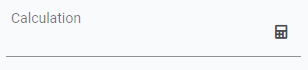Calculations can be as simple or as complex as you need them to be. You can use either mathematics or JavaScript. If you're not familiar with JavaScript, a good source to get started with is W3 Schools.

To calculate variable values, you can reference elements in the form, using the item key, and you can also reference attributes. When you open the calculation tool, these will be listed. Simple click one and it will be added to the calculation.

If only numbers are used in a calculation, the result will be a number. This also requires any attributes you reference to be a number-type or, at least, parsed as a number.

If you use text, the result will a text string, regardless of whether you also use numbers within the calculation.

### Mathematic Calculation

In the following example, we are using a Calculation data type.

In the first screenshot, below, the attribute 'Bottle Volume' will be multiplied by 6. In this example, the value of Bottle Volume is 750.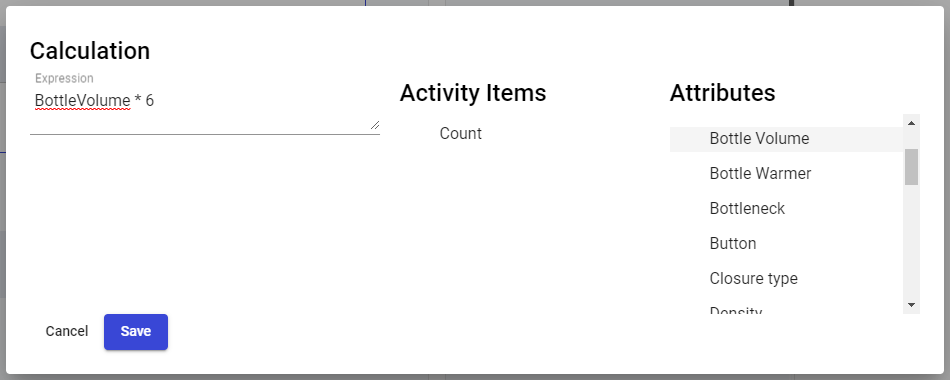The second screenshot is from the activity from the operator's perspective, which is shows the result of the calculation.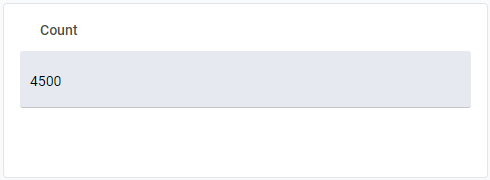### JavaScript Calculation

If you're comfortable using JavaScript in you calculations, you can unlock a lot of extra functionality within TilliT. In the example below, we are using the Read only note data type. The calculation is an optional section of Read only note. You can simply use the Name or Hint property to write your note. However, using the Calculation property allows you to write more dynamic notes.

In the first screenshot, the note is made of 3 parts. The first part is a text string, written within quotations. We then add the attribute Closure Type, which is has a text value, but you can use number and Boolean types as well. Booleans will be parsed as text string (stating true or false).

Finally, we add another text string to finish the statement.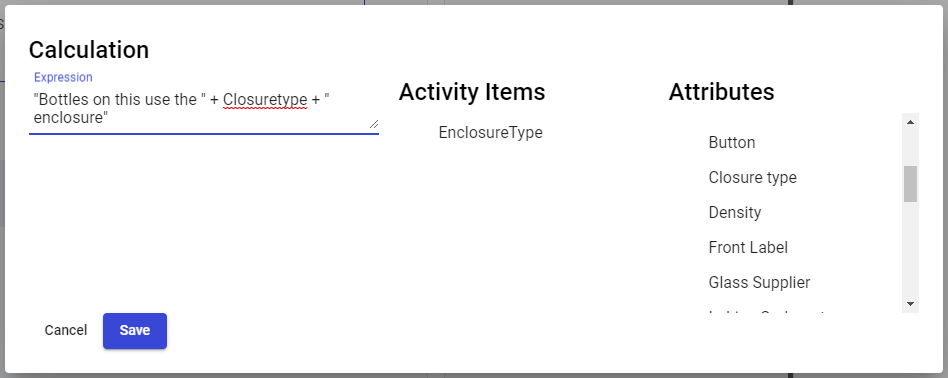In our example, Closure Type has a value of "Screw Cap", so the result of our calculation above becomes: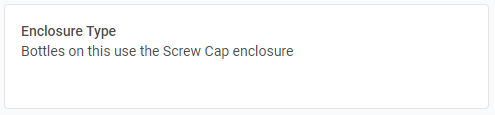This work on a calculation in the same way. The only difference would be a Data Type of Calculation, with the different card in the activity form: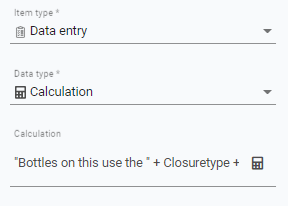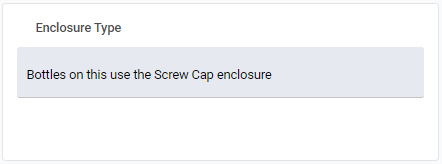Back to: Activity Templates - Details